×#### Thank you for registering.

One of our academic counsellors will contact you within 1 working day.

Click to Chat

1800-1023-196

+91-120-4616500

CART 0

• 0

MY CART (5)

Use Coupon: CART20 and get 20% off on all online Study Material

ITEM
DETAILS
MRP
DISCOUNT
FINAL PRICE
Total Price: Rs.

There are no items in this cart.
Continue Shopping• Complete JEE Main/Advanced Course and Test Series
• OFFERED PRICE: Rs. 15,900
• View Details

```Chapter 14: Coordinate Geometry Exercise – 14.4

Question: 1

Find the centroid of the triangle whose vertices are:

(i) (1, 4),( -1, -1) and (3, -2)

Solution:

We know that the coordinates of the centroid of a triangle whose vertices are

(x1, y1), (x2, y2), (x3, y3) are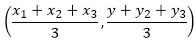So, the coordinates of the centroid of a triangle whose vertices are

(1, 4), (-1, -1) and (3, -2) are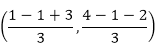Question: 2

Two vertices of a triangle are (1, 2), (3, 5) and its centroid is at the origin. Find the coordinates of the third vertex.

Solution:

Let the coordinates of the third vertex bee (x, y), Then Coordinates of centroid of triangle are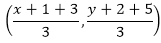We have centroid is at origin (0, 0)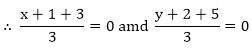⇒ x + 4 = 0 ⇒ y + 7= 0

⇒ x = - 4 ⇒ y = - 7

Question: 3

Prove analytically that the line segment joining the middle points of two sides of a triangle is equal to half of the third side.

Solution: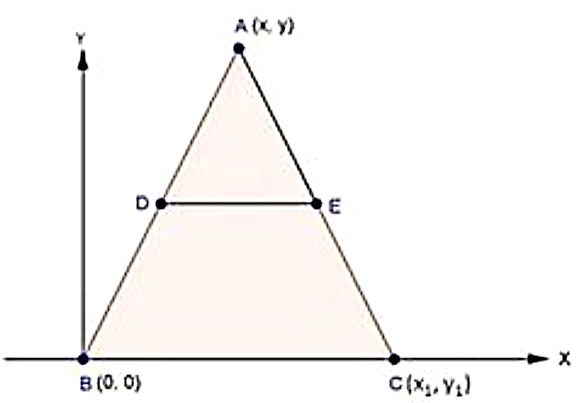Let ABC be a triangle such that BC is along x-axis Coordinates of A, B and C are (x, y), (0, 0) and (x1,y1)

D and E are the mid-points of AB and AC respectively

Coordinates of D areCoordinates of E areQuestion: 4

Prove that the lines joining the middle points of the opposite sides of a quadrilateral and the join of the middle points of its diagonals meet in a point and bisect one another.

Solution:

Let OBCD be the quadrilateral P, Q, R, S be the midpoint off OB, CD, OD and BC. Let the coordinates of 0, B, C, D are (0,0),(x, 0), (x, y) and (0,y)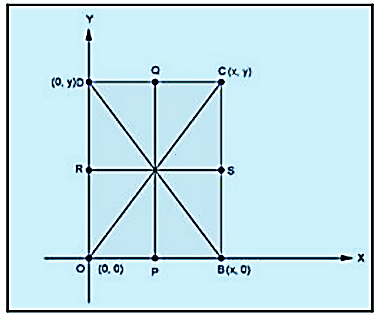Coordinates of P are (x/2, 0)

Coordinates of Q are (x/2, y)

Coordinates of R are (0, y/2)

Coordinates of S are (x, y/2)

Coordinates of midpoint of PQ areCoordinates of midpoint of RS are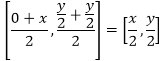Since, the coordinates of the mid-point of PQ = coordinates of mid-point of RS

∴ PQ and RS bisect each other

Question: 6

If G be the centroid of a triangle ABC and P be any other point in the plane, prove that

PA2+ PB2 + PC2 = GA2 + GB2 + GC2 + 3GP2

Solution:

Let A (0, 0), B (a, 0), and C (c, d) are the o-ordinates of triangle ABC

Hence,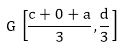i.e.,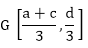let p(x, y)

to Prove:

PA2 + PB2 + PC2 = GA2+ GB2 + GC2 + 3GP2

Or, PA2 + PB2 + PC2 = GA2+ GB2+ GC2+ GP2+ GP2 + GP2

Or, PA2- GP2+ PB2- GP2+ PC2+ GP2 = GA2 + GB2 + GC2

Proof:

PA2 = x2 + y2PB2 = (x - a)2 + y2

P  = (x - c)2 + (y – d)2

L.H.S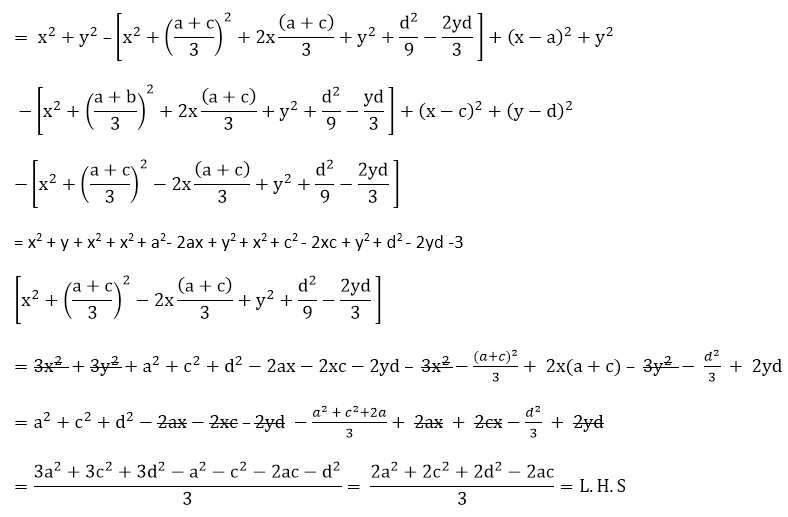Solving R.H.S

GA2+ GB2+ GC2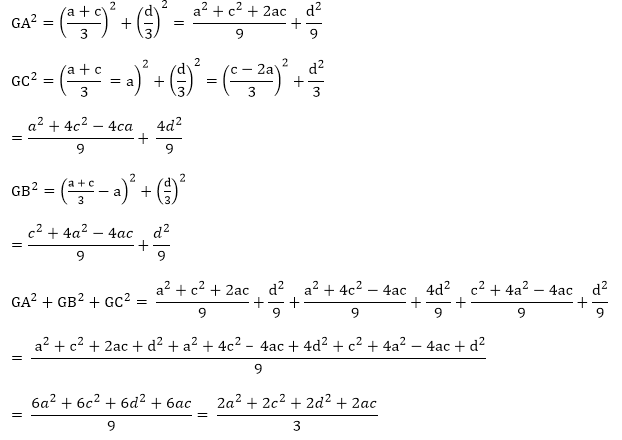∴  L. H. S = R. H. S
```### Course Features

• 728 Video Lectures
• Revision Notes
• Previous Year Papers
• Mind Map
• Study Planner
• NCERT Solutions
• Discussion Forum
• Test paper with Video Solution